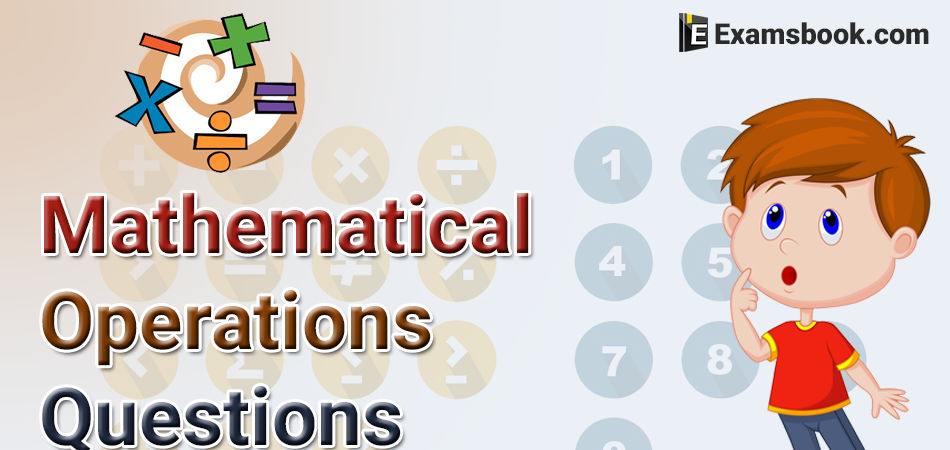• Save

# Mathematical Operations Reasoning Questions with Examples## Mathematical Operations Questions for Competitive Exams

Mathematical operations reasoning questions are very important in the reasoning section for competitive exams. You just need to understand this topic and try to solve it according to given instructions, you will get good marks on the mathematical topic. Here you can understand easily mathematical operations with their types, examples and practice questions.

This section deals with questions on simple mathematical operations. Here, the four fundamental operations - addition, subtraction, multiplication and division and also statements such as less ‘than; 'greater than', 'equal to', 'not equal to', etc. are represented by symbols, different from the usual ones. The questions involving these operations are set using artificial symbols.

In this type of questions, a simple mathematical equation is given and candidates are required to solve these equations with the help of given instruction in contest with BODMAS rule. In dealing with these questions candidates must know the four essential signs with their operative nature i.e., addition (+), subtraction (-), multiplication (×) and division (÷).

### According to its characteristics, mathematical operation may be categorized in the following types:

1. Numerical Operation                2. Symbolic Operation

#### Numerical Operation

In these types of questions, a mathematical equation is given with arithmetical notification. Candidates are required to solve these questions by using arithmetical concepts stated with VBODMAS rule.

Example.1. If + stands for ‘division’, × stands for ‘addition’,  stands for ‘multiplication’ and ÷ stands for subtraction, then which of the following equations is correct?

(a) 36 × 6 + 7 ÷ 2 – 6 = 20

(b) 36 + 6 – 3 × 5 ÷ 3 = 24.

(c) 36 ÷ 6 + 3 × 5 – 3 = 45.

(d) 36 -6 + 3 × 5 ÷ 3 = 74.

Ans. d

Solution

36 – 6 + 3 × 5 ÷ 3

→ 36 × 6 ÷ 3 + 5 – 3.

→ 36 × 2 + 5 – 3 = 74.

→ 72 + 5 – 3 = 74.

#### Symbolic Operation

In these types of questions, a mathematical equation is given with symbolic notification. Candidates are required to replace these symbols according to the given instruction, so that equations may have the perfect mean.

Example. If P denotes ‘+’, Q denotes ‘–‘, R denotes ‘×’ and S denotes ‘÷’, then which of the following statement is correct ?

(a) 16 R 12 P 49 S 7 Q 9 = 200

(b) 32 S 8 R 9 = 160 Q 12 R 12

(c) 8 R 8 P 8 S 8 Q 8 = 57

(d) 36 R 4 S 8 Q 7 P 4 = 10

Ans. c

Solution

= 8 × 8 + 8 ÷ 8 – 8

= 8 × 8 + (8/8) – 8

= 64 +1 – 8 = 57.